# Arithmetic

For the song by Brooke Fraser, see Arithmetic (song).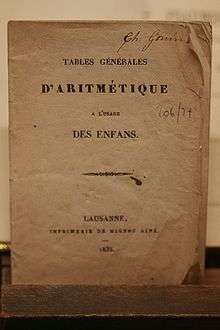Arithmetic tables for children, Lausanne, 1835

Arithmetic or arithmetics (from the Greek ἀριθμός arithmos, "number") is the oldest and most elementary branch of mathematics. It consists of the study of numbers, especially the properties of the traditional operations between them—addition, subtraction, multiplication and division. Arithmetic is an elementary part of number theory, and number theory is considered to be one of the top-level divisions of modern mathematics, along with algebra, geometry, and analysis. The terms arithmetic and higher arithmetic were used until the beginning of the 20th century as synonyms for number theory and are sometimes still used to refer to a wider part of number theory.

## History

The prehistory of arithmetic is limited to a small number of artifacts which may indicate the conception of addition and subtraction, the best-known being the Ishango bone from central Africa, dating from somewhere between 20,000 and 18,000 BC, although its interpretation is disputed.

The earliest written records indicate the Egyptians and Babylonians used all the elementary arithmetic operations as early as 2000 BC. These artifacts do not always reveal the specific process used for solving problems, but the characteristics of the particular numeral system strongly influence the complexity of the methods. The hieroglyphic system for Egyptian numerals, like the later Roman numerals, descended from tally marks used for counting. In both cases, this origin resulted in values that used a decimal base but did not include positional notation. Complex calculations with Roman numerals required the assistance of a counting board or the Roman abacus to obtain the results.

Early number systems that included positional notation were not decimal, including the sexagesimal (base 60) system for Babylonian numerals and the vigesimal (base 20) system that defined Maya numerals. Because of this place-value concept, the ability to reuse the same digits for different values contributed to simpler and more efficient methods of calculation.

The continuous historical development of modern arithmetic starts with the Hellenistic civilization of ancient Greece, although it originated much later than the Babylonian and Egyptian examples. Prior to the works of Euclid around 300 BC, Greek studies in mathematics overlapped with philosophical and mystical beliefs. For example, Nicomachus summarized the viewpoint of the earlier Pythagorean approach to numbers, and their relationships to each other, in his Introduction to Arithmetic.

Greek numerals were used by Archimedes, Diophantus and others in a positional notation not very different from ours. Because the ancient Greeks lacked a symbol for zero (until the Hellenistic period), they used three separate sets of symbols. One set for the unit's place, one for the ten's place, and one for the hundred's. Then for the thousand's place they would reuse the symbols for the unit's place, and so on. Their addition algorithm was identical to ours, and their multiplication algorithm was only very slightly different. Their long division algorithm was the same, and the square root algorithm that was once taught in school was known to Archimedes, who may have invented it. He preferred it to Hero's method of successive approximation because, once computed, a digit doesn't change, and the square roots of perfect squares, such as 7485696, terminate immediately as 2736. For numbers with a fractional part, such as 546.934, they used negative powers of 60 instead of negative powers of 10 for the fractional part 0.934. The ancient Chinese used a similar positional notation. Because they also lacked a symbol for zero, they had one set of symbols for the unit's place, and a second set for the ten's place. For the hundred's place they then reused the symbols for the unit's place, and so on. Their symbols were based on the ancient counting rods. It is a complicated question to determine exactly when the Chinese started calculating with positional representation, but it was definitely before 400 BC. The Bishop of Syria, Severus Sebokht (650 AD), "Indians possess a method of calculation that no word can praise enough. Their rational system of mathematics, or of their method of calculation. I mean the system using nine symbols."

Leonardo of Pisa (Fibonacci) in 1200 AD wrote in Liber Abaci "The method of the Indians (Modus Indoram) surpasses any known method to compute. It's a marvelous method. They do their computations using nine figures and symbol zero".

The gradual development of Hindu–Arabic numerals independently devised the place-value concept and positional notation, which combined the simpler methods for computations with a decimal base and the use of a digit representing 0. This allowed the system to consistently represent both large and small integers. This approach eventually replaced all other systems. In the early 6th century AD, the Indian mathematician Aryabhata incorporated an existing version of this system in his work, and experimented with different notations. In the 7th century, Brahmagupta established the use of 0 as a separate number and determined the results for multiplication, division, addition and subtraction of zero and all other numbers, except for the result of division by 0. His contemporary, the Syriac bishop Severus Sebokht described the excellence of this system as "... valuable methods of calculation which surpass description". The Arabs also learned this new method and called it hesab.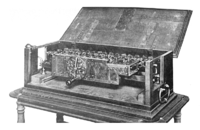Leibniz's Stepped Reckoner was the first calculator that could perform all four arithmetic operations.

Although the Codex Vigilanus described an early form of Arabic numerals (omitting 0) by 976 AD, Fibonacci was primarily responsible for spreading their use throughout Europe after the publication of his book Liber Abaci in 1202. He considered the significance of this "new" representation of numbers, which he styled the "Method of the Indians" (Latin Modus Indorum), so fundamental that all related mathematical foundations, including the results of Pythagoras and the algorism describing the methods for performing actual calculations, were "almost a mistake" in comparison.

In the Middle Ages, arithmetic was one of the seven liberal arts taught in universities.

The flourishing of algebra in the medieval Islamic world and in Renaissance Europe was an outgrowth of the enormous simplification of computation through decimal notation.

Various types of tools have been invented and widely used to assist in numeric calculations. Before Renaissance, they were various types of abaci. More recent examples include slide rules, nomograms and mechanical calculators, such as Pascal's calculator. Presently, they have been supplanted by electronic calculators and computers.

## Arithmetic operations

The basic arithmetic operations are addition, subtraction, multiplication and division, although this subject also includes more advanced operations, such as manipulations of percentages, square roots, exponentiation, and logarithmic functions. Arithmetic is performed according to an order of operations. Any set of objects upon which all four arithmetic operations (except division by 0) can be performed, and where these four operations obey the usual laws, is called a field.

Addition is the basic operation of arithmetic. In its simplest form, addition combines two numbers, the addends or terms, into a single number, the sum of the numbers (Such as 2 + 2 = 4 or 3 + 5 = 8).

Adding more than two numbers can be viewed as repeated addition; this procedure is known as summation and includes ways to add infinitely many numbers in an infinite series; repeated addition of the number 1 is the most basic form of counting.

Addition is commutative and associative so the order the terms are added in does not matter. The identity element of addition (the additive identity) is 0, that is, adding 0 to any number yields that same number. Also, the inverse element of addition (the additive inverse) is the opposite of any number, that is, adding the opposite of any number to the number itself yields the additive identity, 0. For example, the opposite of 7 is −7, so 7 + (−7) = 0.

Addition can be given geometrically as in the following example:

If we have two sticks of lengths 2 and 5, then if we place the sticks one after the other, the length of the stick thus formed is 2 + 5 = 7.

### Subtraction (−)

Main article: Subtraction

Subtraction is the inverse of addition. Subtraction finds the difference between two numbers, the minuend minus the subtrahend. If the minuend is larger than the subtrahend, the difference is positive; if the minuend is smaller than the subtrahend, the difference is negative; if they are equal, the difference is 0.

Subtraction is neither commutative nor associative. For that reason, it is often helpful to look at subtraction as addition of the minuend and the opposite of the subtrahend, that is ab = a + (−b). When written as a sum, all the properties of addition hold.

There are several methods for calculating results, some of which are particularly advantageous to machine calculation. For example, digital computers employ the method of two's complement. Of great importance is the counting up method by which change is made. Suppose an amount P is given to pay the required amount Q, with P greater than Q. Rather than performing the subtraction PQ and counting out that amount in change, money is counted out starting at Q and continuing until reaching P. Although the amount counted out must equal the result of the subtraction PQ, the subtraction was never really done and the value of PQ might still be unknown to the change-maker.

### Multiplication (× or · or *)

Main article: Multiplication

Multiplication is the second basic operation of arithmetic. Multiplication also combines two numbers into a single number, the product. The two original numbers are called the multiplier and the multiplicand, sometimes both simply called factors.

Multiplication may be viewed as a scaling operation. If the numbers are imagined as lying in a line, multiplication by a number, say x, greater than 1 is the same as stretching everything away from 0 uniformly, in such a way that the number 1 itself is stretched to where x was. Similarly, multiplying by a number less than 1 can be imagined as squeezing towards 0. (Again, in such a way that 1 goes to the multiplicand.)

Multiplication is commutative and associative; further it is distributive over addition and subtraction. The multiplicative identity is 1, that is, multiplying any number by 1 yields that same number. Also, the multiplicative inverse is the reciprocal of any number (except 0; 0 is the only number without a multiplicative inverse), that is, multiplying the reciprocal of any number by the number itself yields the multiplicative identity.

The product of a and b is written as a × b or a·b. When a or b are expressions not written simply with digits, it is also written by simple juxtaposition: ab. In computer programming languages and software packages in which one can only use characters normally found on a keyboard, it is often written with an asterisk: a * b.

### Division (÷ or /)

Division is essentially the inverse of multiplication. Division finds the quotient of two numbers, the dividend divided by the divisor. Any dividend divided by 0 is undefined. For distinct positive numbers, if the dividend is larger than the divisor, the quotient is greater than 1, otherwise it is less than 1 (a similar rule applies for negative numbers). The quotient multiplied by the divisor always yields the dividend.

Division is neither commutative nor associative. As it is helpful to look at subtraction as addition, it is helpful to look at division as multiplication of the dividend times the reciprocal of the divisor, that is a ÷ b = a × 1b. When written as a product, it obeys all the properties of multiplication.

## Decimal arithmetic

Decimal representation refers exclusively, in common use, to the written numeral system employing arabic numerals as the digits for a radix 10 ("decimal") positional notation; however, any numeral system based on powers of 10, e.g., Greek, Cyrillic, Roman, or Chinese numerals may conceptually be described as "decimal notation" or "decimal representation".

Modern methods for four fundamental operations (addition, subtraction, multiplication and division) were first devised by Brahmagupta of India. This was known during medieval Europe as "Modus Indoram" or Method of the Indians. Positional notation (also known as "place-value notation") refers to the representation or encoding of numbers using the same symbol for the different orders of magnitude (e.g., the "ones place", "tens place", "hundreds place") and, with a radix point, using those same symbols to represent fractions (e.g., the "tenths place", "hundredths place"). For example, 507.36 denotes 5 hundreds (102), plus 0 tens (101), plus 7 units (100), plus 3 tenths (10−1) plus 6 hundredths (10−2).

The concept of 0 as a number comparable to the other basic digits is essential to this notation, as is the concept of 0's use as a placeholder, and as is the definition of multiplication and addition with 0. The use of 0 as a placeholder and, therefore, the use of a positional notation is first attested to in the Jain text from India entitled the Lokavibhâga, dated 458 AD and it was only in the early 13th century that these concepts, transmitted via the scholarship of the Arabic world, were introduced into Europe by Fibonacci using the Hindu–Arabic numeral system.

Algorism comprises all of the rules for performing arithmetic computations using this type of written numeral. For example, addition produces the sum of two arbitrary numbers. The result is calculated by the repeated addition of single digits from each number that occupies the same position, proceeding from right to left. An addition table with ten rows and ten columns displays all possible values for each sum. If an individual sum exceeds the value 9, the result is represented with two digits. The rightmost digit is the value for the current position, and the result for the subsequent addition of the digits to the left increases by the value of the second (leftmost) digit, which is always one. This adjustment is termed a carry of the value 1.

The process for multiplying two arbitrary numbers is similar to the process for addition. A multiplication table with ten rows and ten columns lists the results for each pair of digits. If an individual product of a pair of digits exceeds 9, the carry adjustment increases the result of any subsequent multiplication from digits to the left by a value equal to the second (leftmost) digit, which is any value from 1 to 8 (9 × 9 = 81). Additional steps define the final result.

Similar techniques exist for subtraction and division.

The creation of a correct process for multiplication relies on the relationship between values of adjacent digits. The value for any single digit in a numeral depends on its position. Also, each position to the left represents a value ten times larger than the position to the right. In mathematical terms, the exponent for the radix (base) of 10 increases by 1 (to the left) or decreases by 1 (to the right). Therefore, the value for any arbitrary digit is multiplied by a value of the form 10n with integer n. The list of values corresponding to all possible positions for a single digit is written as {..., 102, 10, 1, 10−1, 10−2, ...}.

Repeated multiplication of any value in this list by 10 produces another value in the list. In mathematical terminology, this characteristic is defined as closure, and the previous list is described as closed under multiplication. It is the basis for correctly finding the results of multiplication using the previous technique. This outcome is one example of the uses of number theory.

## Compound unit arithmetic

Compound unit arithmetic is the application of arithmetic operations to mixed radix quantities such as feet and inches, gallons and pints, pounds shillings and pence, and so on. Prior to the use of decimal-based systems of money and units of measure, the use of compound unit arithmetic formed a significant part of commerce and industry.

### Basic arithmetic operations

The techniques used for compound unit arithmetic were developed over many centuries and are well-documented in many textbooks in many different languages. In addition to the basic arithmetic functions encountered in decimal arithmetic, compound unit arithmetic employs three more functions:

• Reduction where a compound quantity is reduced to a single quantity, for example conversion of a distance expressed in yards, feet and inches to one expressed in inches.
• Expansion, the inverse function to reduction, is the conversion of a quantity that is expressed as a single unit of measure to a compound unit, such as expanding 24 oz to 1 lb, 8 oz.
• Normalization is the conversion of a set of compound units to a standard form – for example rewriting "1 ft 13 in" as "2 ft 1 in".

Knowledge of the relationship between the various units of measure, their multiples and their submultiples forms an essential part of compound unit arithmetic.

### Principles of compound unit arithmetic

There are two basic approaches to compound unit arithmetic:

• Reduction–expansion method where all the compound unit variables are reduced to single unit variables, the calculation performed and the result expanded back to compound units. This approach is suited for automated calculations. A typical example is the handling of time by Microsoft Excel where all time intervals are processed internally as days and decimal fractions of a day.
• On-going normalization method in which each unit is treated separately and the problem is continuously normalized as the solution develops. This approach, which is widely described in classical texts, is best suited for manual calculations. An example of the ongoing normalization method as applied to addition is shown below.
UK pre-decimal currency
 4 farthings (f) = 1 penny 12 pennies (d) = 1 shilling 20 shillings (s) = 1 pound (£)The addition operation is carried out from right to left; in this case, pence are processed first, then shillings followed by pounds. The numbers below the "answer line" are intermediate results. The total in the pence column is 25. Since there are 12 pennies in a shilling, 25 is divided by 12 to give 2 with a remainder of 1. The value "1" is then written to the answer row and the value "2" carried forward to the shillings column. This operation is repeated using the values in the shillings column, with the additional step of adding the value that was carried forward from the pennies column. The intermediate total is divided by 20 as there are 20 shillings in a pound. The pound column is then processed, but as pounds are the largest unit that is being considered, no values are carried forward from the pounds column. It should be noted that for the sake of simplicity, the example chosen did not have farthings.

### Operations in practice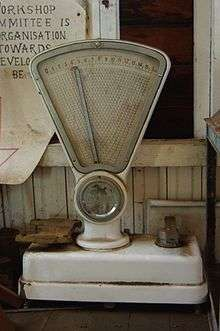A scale calibrated in imperial units with an associated cost display.

During the 19th and 20th centuries various aids were developed to aid the manipulation of compound units, particularly in commercial applications. The most common aids were mechanical tills which were adapted in countries such as the United Kingdom to accommodate pounds, shillings, pennies and farthings and "Ready Reckoners" – books aimed at traders that catalogued the results of various routine calculations such as the percentages or multiples of various sums of money. One typical booklet that ran to 150 pages tabulated multiples "from one to ten thousand at the various prices from one farthing to one pound".

The cumbersome nature of compound unit arithmetic has been recognized for many years – in 1586, the Flemish mathematician Simon Stevin published a small pamphlet called De Thiende ("the tenth") in which he declared that the universal introduction of decimal coinage, measures, and weights to be merely a question of time while in the modern era, many conversion programs, such as that embedded in the calculator supplied as a standard part of the Microsoft Windows 7 operating system display compound units in a reduced decimal format rather than using an expanded format (i.e. "2.5 ft" is displayed rather than "2 ft 6 in").

## Number theory

Main article: Number theory

Until the 19th century, number theory was a synonym of "arithmetic". The addressed problems were directly related to the basic operations and concerned primality, divisibility, and the solution of equations in integers, such as Fermat's last theorem. It appeared that most of these problems, although very elementary to state, are very difficult and may not be solved without very deep mathematics involving concepts and methods from many other branches of mathematics. This led to new branches of number theory such as analytic number theory, algebraic number theory, Diophantine geometry and arithmetic algebraic geometry. Wiles' proof of Fermat's Last Theorem is a typical example of the necessity of sophisticated methods, which go far beyond the classical methods of arithmetic, for solving problems that can be stated in elementary arithmetic.

## Arithmetic in education

Primary education in mathematics often places a strong focus on algorithms for the arithmetic of natural numbers, integers, fractions, and decimals (using the decimal place-value system). This study is sometimes known as algorism.

The difficulty and unmotivated appearance of these algorithms has long led educators to question this curriculum, advocating the early teaching of more central and intuitive mathematical ideas. One notable movement in this direction was the New Math of the 1960s and 1970s, which attempted to teach arithmetic in the spirit of axiomatic development from set theory, an echo of the prevailing trend in higher mathematics.

Also, arithmetic was used by Islamic Scholars in order to teach application of the rulings related to Zakat and Irth. This was done in a book entitled The Best of Arithmetic by Abd-al-Fattah-al-Dumyati.

The book begins with the foundations of mathematics and proceeds to its application in the later chapters.

## Notes

1. "Mathematics". Science Clarified. Retrieved 23 October 2012.
2. Davenport, Harold, The Higher Arithmetic: An Introduction to the Theory of Numbers (7th ed.), Cambridge University Press, Cambridge, UK, 1999, ISBN 0-521-63446-6.
3. Rudman, Peter Strom (2007). How Mathematics Happened: The First 50,000 Years. Prometheus Books. p. 64. ISBN 978-1-59102-477-4.
4. The Works of Archimedes, Chapter IV, Arithmetic in Archimedes, edited by T.L. Heath, Dover Publications Inc, New York, 2002.
5. Joseph Needham, Science and Civilization in China, Vol. 3, page 9, Cambridge University Press, 1959.
6. Reference: Revue de l'Orient Chretien by François Nau pp.327-338. (1929)
7. Reference: Sigler, L., "Fibonacci's Liber Abaci", Springer, 2003.
8. Tapson, Frank (1996). The Oxford Mathematics Study Dictionary. Oxford University Press. ISBN 0 19 914551 2.
9. Leonardo Pisano - page 3: "Contributions to number theory". Encyclopædia Britannica Online, 2006. Retrieved 18 September 2006.
10. Walkingame, Francis (1860). "The Tutor's Companion; or, Complete Practical Arithmetic" (PDF). Webb, Millington & Co. pp. 24–39.
11. Palaiseau, JFG (October 1816). Métrologie universelle, ancienne et moderne: ou rapport des poids et mesures des empires, royaumes, duchés et principautés des quatre parties du monde [Universal, ancient and modern metrology: or report of weights and measurements of empires, kingdoms, duchies and principalities of all parts of the world] (in French). Bordeaux. Retrieved October 30, 2011.
12. Jacob de Gelder (1824). Allereerste Gronden der Cijferkunst [Introduction to Numeracy] (in Dutch). 's-Gravenhage and Amsterdam: de Gebroeders van Cleef. pp. 163–176. Retrieved March 2, 2011.
13. Malaisé, Ferdinand (1842). Theoretisch-Praktischer Unterricht im Rechnen für die niederen Classen der Regimentsschulen der Königl. Bayer. Infantrie und Cavalerie [Theoretical and practical instruction in arithmetic for the lower classes of the Royal Bavarian Infantry and Cavalry School] (in German). Munich. Retrieved 20 March 2012.
14. Encyclopædia Britannica, Vol I, Edinburgh, 1772, Arithmetick
15. Walkingame, Francis (1860). "The Tutor's Companion; or, Complete Practical Arithmetic" (PDF). Webb, Millington & Co. pp. 43–50.
16. Thomson, J (1824). The Ready Reckoner in miniature containing accurate table from one to the thousand at the various prices from one farthing to one pound. Montreal. Retrieved 25 March 2012.
17. Mathematically Correct: Glossary of Terms
18. al-Dumyati, Abd-al-Fattah Bin Abd-al-Rahman al-Banna (1887). "The Best of Arithmetic". World Digital Library (in Arabic). Retrieved 30 June 2013.

## ReferencesLook up arithmetic in Wiktionary, the free dictionary.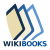Wikibooks has more on the topic of: ArithmeticWikimedia Commons has media related to Arithmetic.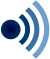Wikiquote has quotations related to: Arithmetic